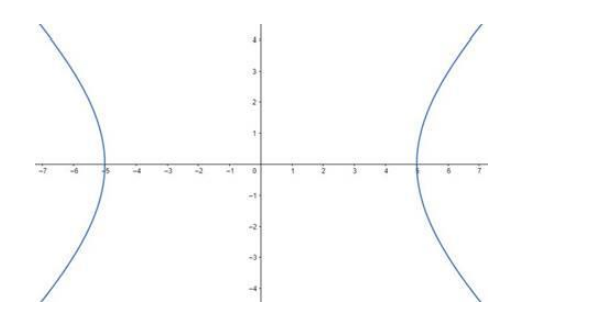# Find the equation of the hyperbola whose foci are

Question:

Find the equation of the hyperbola whose foci are $(\pm 3 \sqrt{5}, 0)$ and the length of the latus rectum is 8 units.

Solution:

Given: Foci are $(\pm 3 \sqrt{5}, 0)$ the length of the latus rectum is 8 units

Need to find: The equation of the hyperbola.

Let, the equation of the hyperbola be:

$\frac{x^{2}}{a^{2}}-\frac{y^{2}}{b^{2}}=1$

The length of the latus rectum is 8 units.

Therefore, $\frac{2 b^{2}}{a}=8 \Rightarrow b^{2}=4 a \cdots$ (1)

The foci are given at $(\pm 3 \sqrt{5}, 0)$That means, ae $=3 \sqrt{5}$, where e is the eccentricity.

We know that

$e=\sqrt{1+\frac{b^{2}}{a^{2}}}$

Therefore

$\Rightarrow \sqrt{1+\frac{b^{2}}{a^{2}}}=3 \sqrt{5}$

$\Rightarrow \quad \frac{\sqrt{a^{2}+b^{2}}}{a}=3 \sqrt{5}$

$\Rightarrow \mathrm{a}^{2}+\mathrm{b}^{2}=45$ [Squaring both sides]

$\Rightarrow \mathrm{a}^{2}+4 \mathrm{a}=45[$ From $(1)]$

$\Rightarrow \mathrm{a}^{2}+4 \mathrm{a}-45=0$

$\Rightarrow \mathrm{a}^{2}+9 \mathrm{a}-5 \mathrm{a}-45=0$

$\Rightarrow(\mathrm{a}+9)(\mathrm{a}-5)=0$

So, either $a=5$ or, $a=-9$

That means, either $b=2 \sqrt{5}$ or, $b=\sqrt{-36}$

The value of $b=\sqrt{-36}$ is not a valid one. So, the $b$ value and its corresponding $a$ value is not acceptable.

Hence, the acceptable value of a is 5 and b is

$2 \sqrt{5}$

So, the equation of the hyperbola is

$\frac{x^{2}}{a^{2}}-\frac{y^{2}}{b^{2}}=1 \Rightarrow \frac{x^{2}}{25}-\frac{y^{2}}{20}=1$ [Answer]